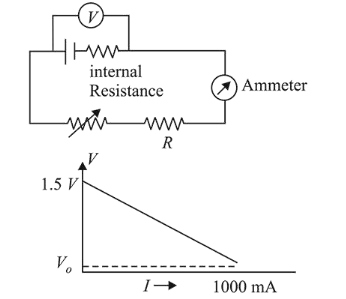# To verify Ohm's law, a student connects the voltmeter across the battery as, shown in the figure.

Question:

To verify Ohm's law, a student connects the voltmeter across the battery as, shown in the figure. The measured voltage is plotted as a function of the current, and the following graph is obtained :If $\mathrm{V}_{\mathrm{o}}$ is almost zero, identify the correct statement:

1. (1) The emf of the battery is $1.5 \mathrm{~V}$ and its internal resistance is $1.5 \Omega$

2. (2) The value of the resistance $\mathrm{R}$ is $1.5 \Omega$

3. (3) The potential difference across the battery is $1.5 \mathrm{~V}$ when it sends a current of $1000 \mathrm{~mA}$

4. (4) The emf of the battery is $1.5 \mathrm{~V}$ and the value of $\mathrm{R}$ is $1.5 \Omega$

Correct Option: 1

Solution:

(1)

When $i=0, V=\varepsilon=1.5$ volt